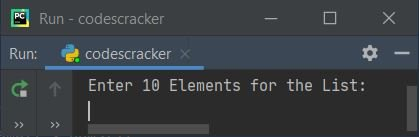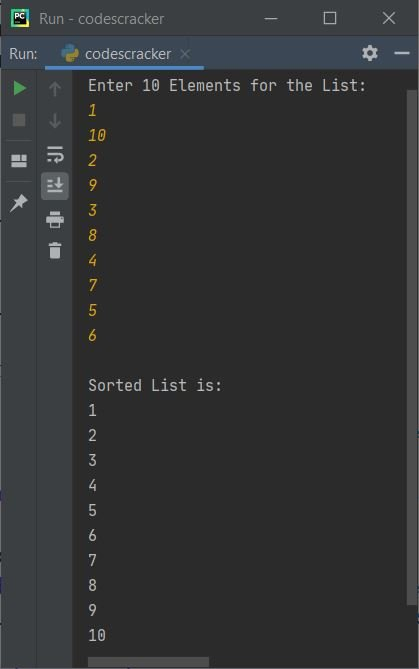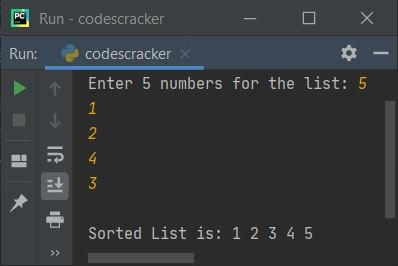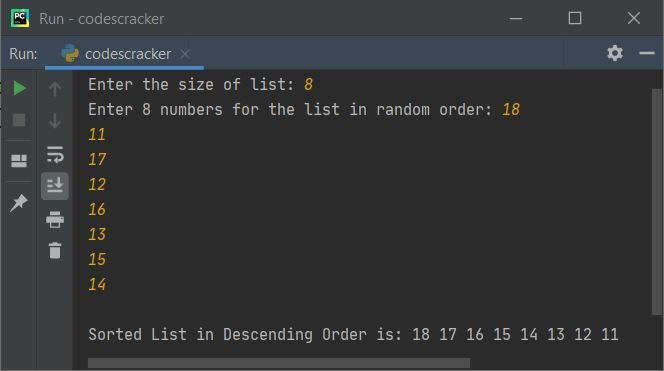# Selection Sort Program in Python

• Selection sort program based on limited elements in list
• Selection sort program based on n elements
• Selection sort program to sort elements of list in descending order

But before going through these programs, if you're not aware about the algorithm or technique used in selection sort, refer to Selection Sort Algorithm and Example to get every required things about it. Now let's move on and create these selection sort programs.

## Selection Sort Program

The question is, write a Python program to sort all elements of a list using selection sort. The program given below is answer to this question:

```nums = []
print("Enter 10 Elements for the List: ")
for i in range(10):
nums.append(int(input()))

for i in range(9):
chk = 0
small = nums[i]
for j in range(i+1, 10):
if small > nums[j]:
small = nums[j]
chk = chk + 1
index = j
if chk != 0:
temp = nums[i]
nums[i] = small
nums[index] = temp

print("\nSorted List is: ")
for i in range(10):
print(nums[i])```

Here is its sample run. The snapshot given below shows the initial output produced by above Python program:Now provide the input say 1, 10, 2, 9, 3, 8, 4, 7, 5, 6 as ten randomly ordered elements for the list, and press `ENTER` key to sort all these elements in ascending order using selection sort technique. The snapshot given below shows the output produced by above program after providing exactly same inputs:## Selection Sort based on n Elements

This program allows user to define the size of list along with its elements. So we can say, this is basically the modified version of previous program. Rest of the things are almost similar to previous program.

```nums = []
print("Enter the size of list: ", end="")
tot = int(input())
print("Enter", tot, "numbers for the list: ", end="")
for i in range(tot):
nums.append(int(input()))

for i in range(tot-1):
chk = 0
small = nums[i]
for j in range(i+1, tot):
if small > nums[j]:
small = nums[j]
chk = chk + 1
index = j
if chk != 0:
temp = nums[i]
nums[i] = small
nums[index] = temp

print("\nSorted List is: ", end="")
for i in range(tot):
print(nums[i], end=" ")```

Here is its sample run with user inputs, 5 as size and 5, 1, 2, 4, 3 as five elements:## Selection Sort in Descending Order

To sort list items in descending order using selection sort technique, just replace the following if:

`if small > nums[j]:`

from above program, that sorts list items in ascending order using selection sort technique, with following if:

`if small < nums[j]:`

As you can see, only a matter of less than (<) and greater than (>) sign, the whole program gets reversed. Here is the complete version of the program:

```nums = []
print("Enter the size of list: ", end="")
tot = int(input())

print("Enter", tot, "numbers for the list in random order: ", end="")
for i in range(tot):
nums.append(int(input()))

for i in range(tot-1):
chk = 0
small = nums[i]
for j in range(i+1, tot):
if small < nums[j]:
small = nums[j]
chk = chk + 1
index = j
if chk != 0:
temp = nums[i]
nums[i] = small
nums[index] = temp

print("\nSorted List in Descending Order is: ", end="")
for i in range(tot):
print(nums[i], end=" ")```

Here is its sample run with user input, 8 as size and 18, 11, 17, 12, 16, 13, 15, 14 as eight elements or numbers:Python Online Test

« Previous Program Next Program »